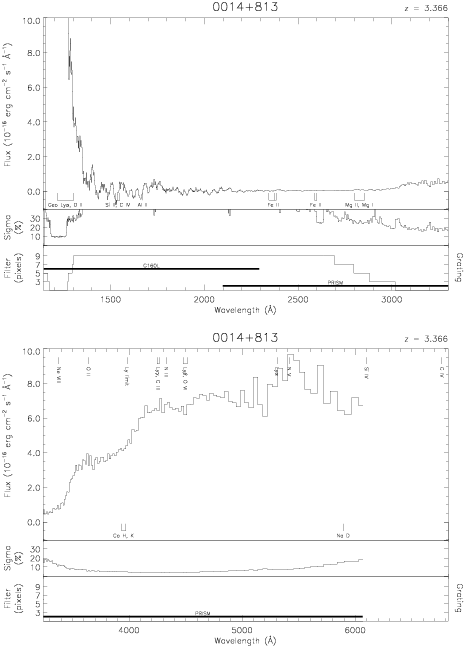Download dataset
TELESCOP= 'HST     '
INSTRUME= 'FOS     '
EQUINOX =               2000.0
DATE-OBS= '1991-06-06T12:35:11'
RA_PNT  =         4.2857663396
DEC_PNT =        81.5858923426
PA_PNT  =        98.3597700000
OBJECT  = '0014+813'
ROOTNM01= 'Y0LF0103T'
GRNDMD01= 'SPECTROSCOPY'
DETECT01= 'BLUE    '
APERID01= 'A-1     '
FGWAID01= 'L15     '
ROOTNM02= 'Y0LF0104T'
GRNDMD02= 'SPECTROSCOPY'
DETECT02= 'BLUE    '
APERID02= 'A-1     '
FGWAID02= 'L15     '
ROOTNM03= 'Y0LF0105T'
GRNDMD03= 'SPECTROSCOPY'
DETECT03= 'BLUE    '
APERID03= 'A-1     '
FGWAID03= 'L15     '
ROOTNM04= 'Y0LF0106T'
GRNDMD04= 'SPECTROSCOPY'
DETECT04= 'BLUE    '
APERID04= 'A-1     '
FGWAID04= 'PRI     '